# Javascript calculators for electronic circuit design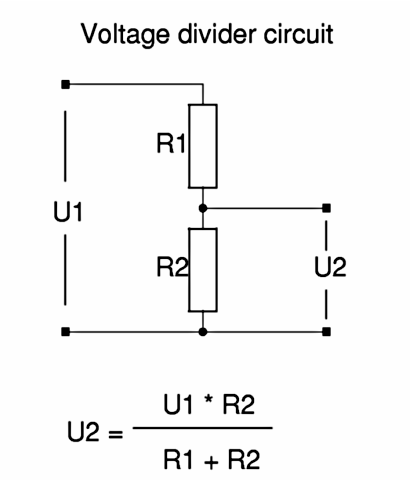## Voltage divider calculator

Enter 3 values and the calculator will calculate the 4th.

All resistors and all voltages must be of the same units.
Example: Enter voltages in Volt or all voltages in mV. Enter all resistors in Ohm or all in KOhm. Do not mix units. Enter only numbers without any letters (units).

 R1: | U1: R2: U2: |

If there is a load applied on the output (U2) then use for R2 the equivalent resistor which consists of the actual R2 value and the load resistor.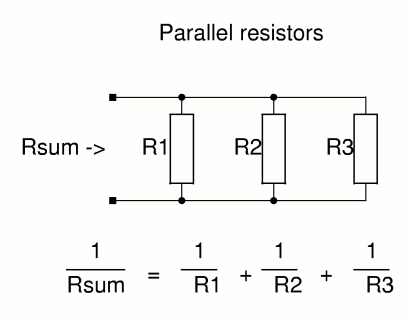## Calculate the equivalent resistor for a number of parallel resistors

This calculator calculates the equivalent resistor (Rsum) of two parallel resistors or three parallel resistors. If you have only two resistors then enter only two values and leave the other blank. Enter only numbers without any letters (units).

 R1: || R2: || R3: = Rsum: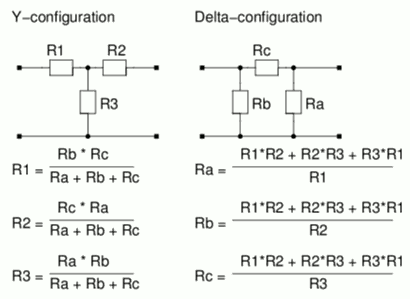## y-Δ transform (Wye-Delta conversion)

This calculator can be used to transform resistors in Y-configuration into the equivalent delta-configuration.

 R1: R2: => Rc: R3: Rb: Ra:

## Δ-y transform (Delta-Wye conversion)

This calculator can be used to transform resistors in Delta-configuration into the equivalent Y-configuration.

 Rc: => R1: R2: Rb: Ra: R3: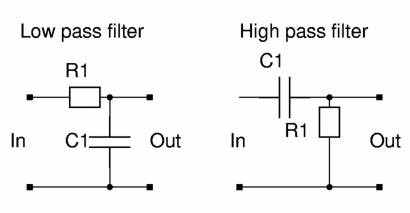## First order RC-filter (high/low pass filter)

The cut-off frequency is defined as the frequency at which the voltage at the output of the filter is 3dB below the input. In other words the output signal is 70.7% or 1/sqrt(2) below the input signal.

For a first order RC-filter the cut-off frequency (fc) is calculated as follows:
R1 * C1 = 1/ω = 1/ (2 π fc)

The time constant ω is equivalent to 2 π fc. Engineers prefer to use fc while many scientists use ω.

Enter 2 values and the calculator will calculate the 3rd value. Select the correct units.

 R1: KOhm Ohm MOhm C1: nF µF pF fc: KHz MHz Hz

## Ohm's Law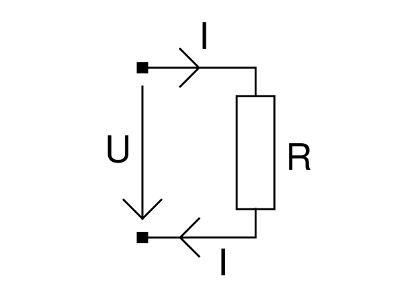Symbols: U=Voltage [Volt,V], I=Current [Ampere,A], R=Resistance [Ohm,Ω]

R=U/I
U=R*I
I=U/R

Simple general purpose calculator:
 = Operators: + - * / ^ Functions: sqrt() sqr() pow(,) Usage: Enter the plain numbers without units. calculate four times two: enter 4*2 in the yellow box and press calculate. calculate 3.22: enter sqr(3.2) in the yellow box and press calculate.

## Z-values of coils/capacitors/resistors

Coil: Z = jωL
Capacitor: Z = - j(1/ωC)
Resistor: Z = R

with ω = 2 π f
j is the imaginary part of the complex number also known as "i"
The unit of Z is Ohm (Ω)

## Electric power consumption

Symbols: U=Voltage [Volt,V], I=Current [Ampere,A], R=Resistance [Ohm,Ω], P=Power [Watt,W]

The power consumtion (P) of an electic device with the resistance R, current I and voltage U is:

P=U*I
P=U2/R
P=I2*R

If R is a simple resistor (and e.g not a motor) then all power will be dissipated as heat.

You can use the general purpose calclulator under "Ohm's Law" for this formula.

This page is operting system independent. It only requires a javascript capable webbrowser. You can install it locally on your PC just by saving this html page.

© Guido Socher , version: 2022-09-30
History: 2009-06-28 -- first version, 2013-03-09 added general purpose calc for ohm's law, 2018-05-19 -- inline images just one page, 2022-09-30 delta-y conversions